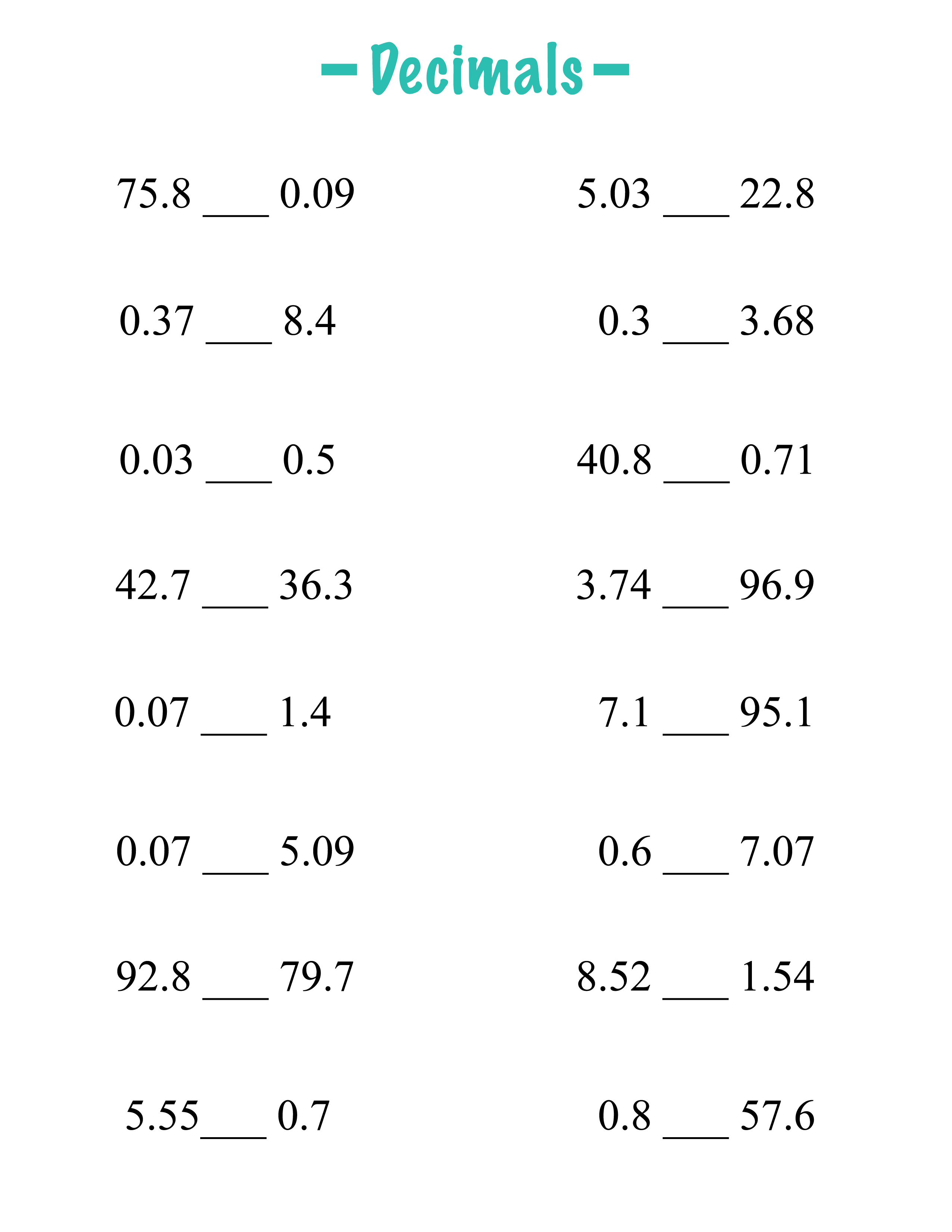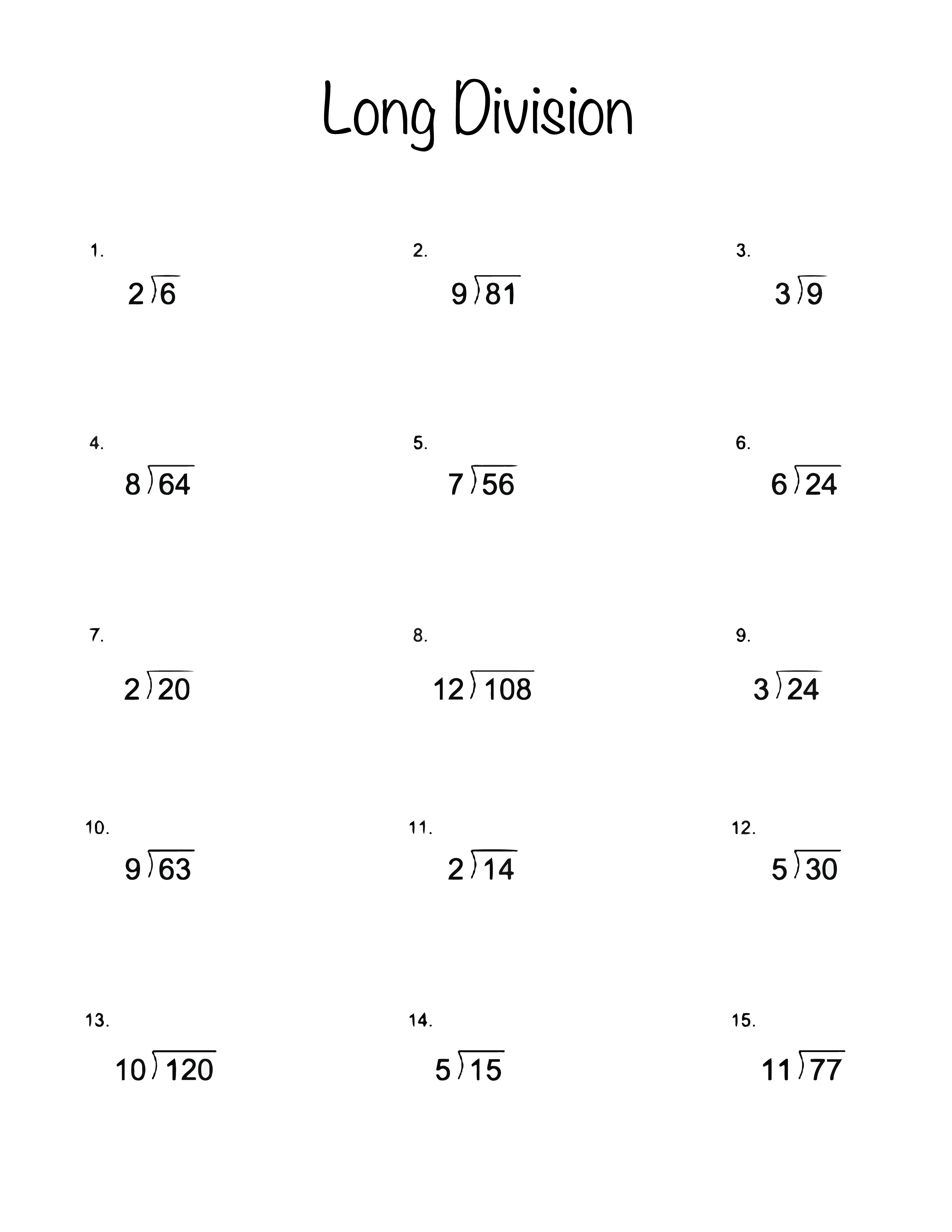After learning all the 3rd grade math, it’s time to move onto 4th grade math! If you want additional worksheets, check out our post here.

In past worksheets, students focused on whole numbers. In this post, we’ll expand their skills into fractions and decimals (as well as continuing to work on their multiplication and division skills). These numbers will come in useful in more complicated mathematical equations as they get older!

## Fraction worksheet

Fractions can look scary, but an introduction to them doesn’t have to be! You can explain to your students that fractions are just a smaller part of the numbers they’ve already been working with. Fractions are always build in relation to the whole number. In this worksheet, students will be adding fractions with the same denominator (the bottom number of the fraction). If the top number (the numerator) becomes more than the denominator, students should rewrite the answer as a mixed number (the whole number and the fraction). For example, the first answer on the second line would be 8/7, or 1 and 1/7.## Decimals

Decimals are another expression of fractions. In this worksheet, students will use the less than (<) or greater than (>) symbol to compare the two decimals. This will give students the chance to learn more about decimals and how they work. For instance, the first answer would look like 75.8 > 0.09## Multiplication

We know that as kids get older, worksheets get more boring. We don’t want that to happen too soon here on Printer Friendly! Here we have a downloadable worksheet that involves some coloring. This worksheet expands multiplication skills, but also allows kids to use some markers or colored pencils to play around.## Long division

Long division is another skill 4th graders will be practicing. We understand that many adults don’t remember how to do long division (I certainly don’t!) so if you need help explaining long division, check out this post.